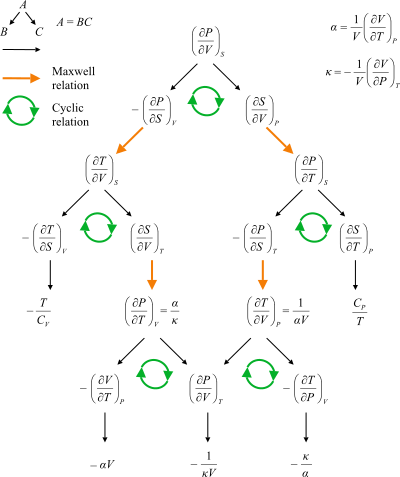# Maxwell relations

For electromagnetic equations, see Maxwell's equations.Flow chart showing the paths between the Maxwell relations. P: pressure, T: temperature, V: volume, S: entropy, α: coefficient of thermal expansion, κ: compressibility, CV: heat capacity at constant volume, CP: heat capacity at constant pressure.

Maxwell's relations are a set of equations in thermodynamics which are derivable from the symmetry of second derivatives and from the definitions of the thermodynamic potentials. These relations are named for the nineteenth-century physicist James Clerk Maxwell.

## Equations

The structure of Maxwell relations is a statement of equality among the second derivatives for continuous functions. It follows directly from the fact that the order of differentiation of an analytic function of two variables is irrelevant (Schwarz theorem). In the case of Maxwell relations the function considered is a thermodynamic potential and xi and xj are two different natural variables for that potential:

 Schwarz' theorem (general)where the partial derivatives are taken with all other natural variables held constant. It is seen that for every thermodynamic potential there are n(n − 1)/2 possible Maxwell relations where n is the number of natural variables for that potential.

## The four most common Maxwell relations

The four most common Maxwell relations are the equalities of the second derivatives of each of the four thermodynamic potentials, with respect to their thermal natural variable (temperature T; or entropy S) and their mechanical natural variable (pressure P; or volume V):

 Maxwell's relations (common)where the potentials as functions of their natural thermal and mechanical variables are the internal energy U(S, V), enthalpy H(S, P), Helmholtz free energy F(T, V) and Gibbs free energy G(T, P). The thermodynamic square can be used as a mnemonic to recall and derive these relations. The usefulness of these relations lies in their quantifying entropy changes, which are not directly measurable, in terms of measurable quantities like temperature, volume, and pressure.

### Derivation

Maxwell relations are based on simple partial differentiation rules, in particular the total differential of a function and the symmetry of evaluating second order partial derivatives.

## General Maxwell relationships

The above are not the only Maxwell relationships. When other work terms involving other natural variables besides the volume work are considered or when the number of particles is included as a natural variable, other Maxwell relations become apparent. For example, if we have a single-component gas, then the number of particles N  is also a natural variable of the above four thermodynamic potentials. The Maxwell relationship for the enthalpy with respect to pressure and particle number would then be:where μ is the chemical potential. In addition, there are other thermodynamic potentials besides the four that are commonly used, and each of these potentials will yield a set of Maxwell relations.

Each equation can be re-expressed using the relationshipwhich are sometimes also known as Maxwell relations.